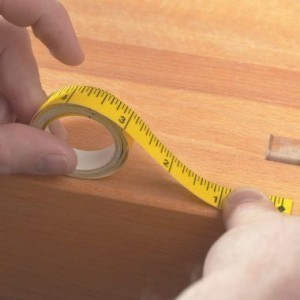# Imperial Measurements

Considered as a system of units, imperial measurements aresometimes referred to as the imperial system or imperial units. They were first defined as part of the 1824 British Weights and Measures Act. They were refined and reduced since 1959. After that, these measurements were officially used throughout the British Empire. In the late 20th century, countries under this former empire decided to adopt the metric system instead as their official measurement system. Aside from these important facts, here are some other valuable details about imperial measurements.

### The Different Imperial Units

Imperial measurements are commonly used to describe four major aspects, namely the mass, volume, area as well as length of objects. For the mass of objects, the small unit is a called a grain, the equivalent value of which is 0.065 grams. The next unit is called a drachm, the value of which is 1.772 grams. In addition, the value of an ounce is 28.349 grams, a pound is 453.592 grams and a stone is 6,350.293 grams. Meanwhile, the equivalent value of a quarter is 12.701 kilograms, a hundredweight is 50.802 kilograms and a ton is 1,016.047 kilograms.

For the volume, the smallest unit is called a fluid ounce, the equivalent value of which is 1 imperial ounce or 0.961 U.S. ounce. Next to this unit is called a gill, the value of which is 5 imperial ounces or 4.804 U.S. ounces. The other units under this system are the pint, the quart and the gallon, the corresponding values of which are 20 imperial ounces or 19.215 U.S. ounces, 40 imperial ounces or 38.430 U.S. ounces and 160 imperial ounces or 153.72 U.S. ounces.

For the area, the units are the perch, rood and acre. The equivalent of each perch is 272.25 square feet, a rood is 10,890 square feet while an acre is 43,560 square feet. For the length, the first three units are the thou, inch and foot. The value of each thou is 0.0254 millimeter, an inch is 25.4 millimeters while a foot is 304.8 millimeters. The next four units are the yard, furlong, mile and league. A yard is equivalent to 914.4 millimeters, a furlong is 201.168 meters, a mile is 1,609.344 meters and a league is 4,828.032 meters.

### Additional Facts and Other Interesting Details

The different imperial measurements also include a number of maritime units. The first one is called a fathom, the equivalent of which is 6 feet. Next to this is a cable, which measures about a tenth of a nautical mile. To measure distances at sea, a nautical mile is commonly used. Its value is the same as 10 cables. Moreover, imperial measurements also include Gunter’s survey units, which are the link, pole and chain.